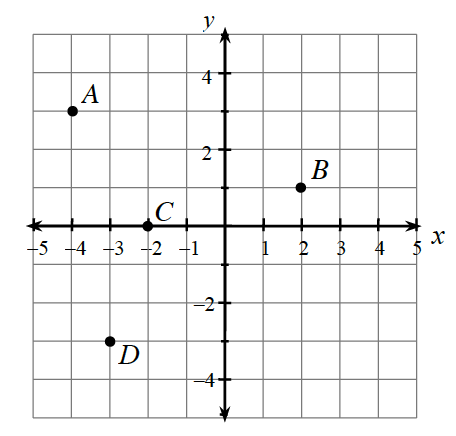### Home > CC3 > Chapter 2 > Lesson 2.1.2 > Problem2-21

2-21.Examine the graph at right.

1. Name the coordinates of points $A$, $B$, $C$, and $D$ in $(x,y)$ form.

Find the change in $x$ and $y$ from the point $(0,0)$.

Point $A$ goes to the left $4$ spaces and up $3$ spaces. How do you write that in $(x,y)$ form?

$A$ is $(−4,3)$. Now find points $B$, $C$, and $D$.

2. Draw a set of axes like the ones shown at right on graph paper.  Then plot points $E(5,2)$, $F(-3,-1)$, $G(0,-4)$, and $H(2,-3)$.

Use the points above and the graph above to plot points $E$, $F$, $G$, and $H$. Remember that the horizontal axis is the $x$-axis, and the vertical axis is the $y$-axis.

https://www.desmos.com/calculator/qd9opgyjnehttps://www.desmos.com/calculator/qd9opgyjneUse the eTool below to input the points into the table and graph the results.
Click the link at right for the full version of the eTool: 2-21 HW eTool